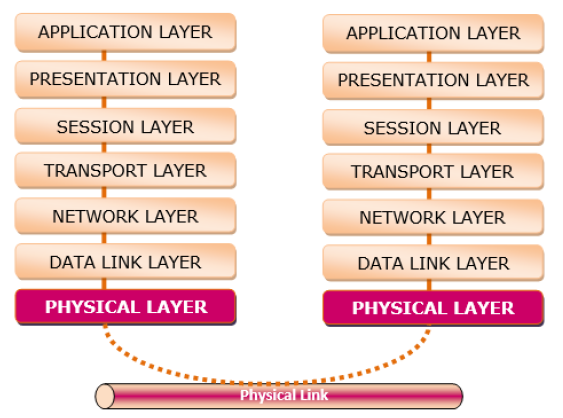# The Physical Layer of OSI Model

Computer NetworkComputer EngineeringMCA

#### Artificial Neural Network and Machine Learning using MATLAB

54 Lectures 4 hours

The physical layer (Layer 1) deals with transmission of individual bits from one node to another over a physical medium.

The main functions of the physical layer are as follows −

• It defines the physical characteristics and functions of the physical devices and interfaces so that transmission can occur. It states the number of pins in each of the devices as well as the purpose of each pin.
• It lays out the transmission medium and type of signal for transmitting the bits, i.e., electrical pulses, optical pulses or radio signals.
• It defines the procedure of encoding of the bits, for example, how many volts should represent a 0 bit and 1 bit in case of electrical signals.
• It states the data transmission rate, i.e., number of bits transmitted per second; and the duration of a bit, i.e., how long a bit stays.
• It defines the topology, i.e., physical layout, of the network devices.
• It also states the direction of transmission, i.e., whether the transmission is in simplex mode, half-duplex mode or full-duplex mode.